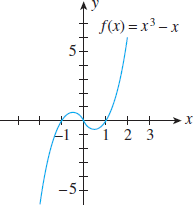Meena A.

# Graph and limits

TANAPCALC10 2.4.064.

My Notes

Use the graph of the function f to determine the following limits, if they exist. (If an answer does not exist, enter DNE.)The x y-coordinate plane is given. The curve labeled f(x) = x3 − x enters the window in the third quadrant, goes up and right becoming less steep, crosses the x-axis at x = −1, changes direction at the approximate point (−0.58, 0.4),goes down and right becoming more steep, passes through the origin, goes down and right becoming less steep, changes direction at the approximate point (0.58, −0.4), goes up and right becoming more steep, crosses the x-axis at x = 1, and exits the window in the first quadrant.

lim x→∞ f(x)

=lim x→−∞ f(x)

=